## What is Mean or Average?

Phillips (2000) defined the mean of two real positive numbers,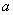and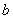as a mapping or function of the two numbers into a real positive number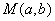. The mapping must satisfy three properties: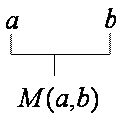1. If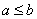then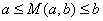. The mean value always lies between the two numbers.
2.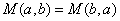. Mean is symmetric inand3. If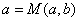then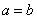Other properties of Mean:

4. Homogeneous mean will satisfy one additional property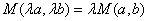. Examples of homogeneous mean are arithmetic mean, geometric mean and harmonic mean.

5. If, then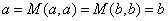. This property happens because of the first property. Note that the property number 3 is the exact contrary of this property number 5.

Expanding for more than two numbers, I may generalize the three properties of mean and the definition of mean. Mean is defined as a mapping or function of the real positive numbers into a real positive number that satisfy property that the mean value always lies between the input numbers .

Three Properties that defined Mean:

1. If we have numbers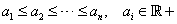, then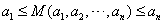2. The order of input numbers is not important. Mean is symmetric in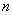dimension. Example: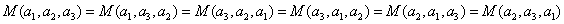3. If all inputs are equal, then the mean value is equal to any one of its input. From property number 1 we can deduce that if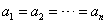then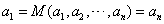Note:

1. Mean is defined only for real positive number input. No negative numbers is allowed as input
2. The number of input is finite up to(not infinite)
3. The mean value is somewhere in between the lowest and the highest input numbers. The mean value will not go outside this range of input.
4. Actually, the symmetric property of mean is not a necessary condition. Others may define unsymmetrical mean.

Preferable reference for this tutorial is

Teknomo, Kardi (2015) Mean and Average. https:\\people.revoledu.com\kardi\tutorial\BasicMath\Average\